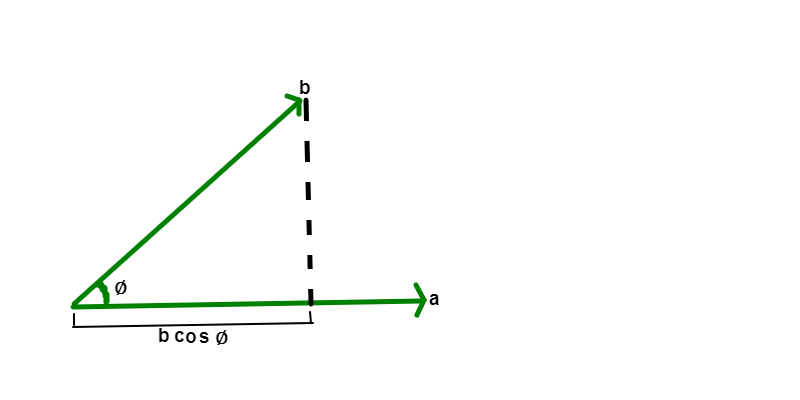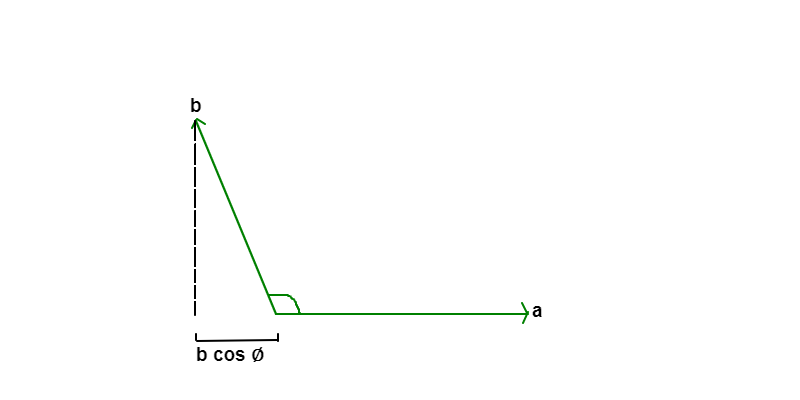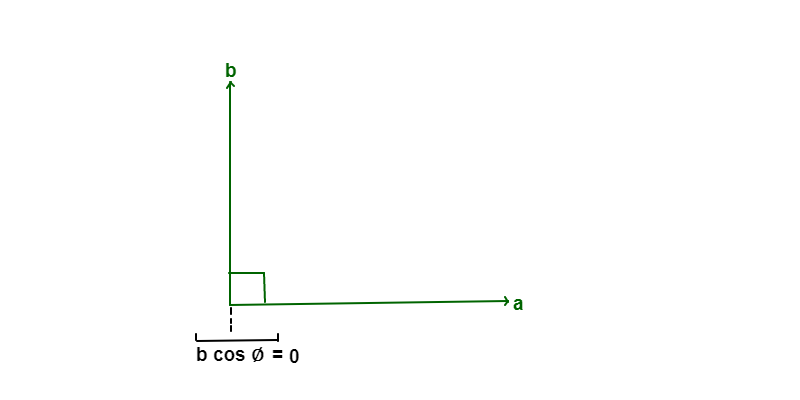GFG App
Open AppBrowser
Continue

Two vectors or a vector and a scalar can be multiplied. There are mainly two kinds of products of vectors in physics, scalar multiplication of vectors and Vector Product (Cross Product) of two vectors. The result of the scalar product of two vectors is a number (a scalar). The common use of the scalar product is to define work and energy relations like to find the work performed on an object by a force (vector) while causing the displacement of the object, this work can be defined as a scalar product of the force vector with the displacement vector.

Scalar and Vector Quantities are defined as:

• Scalars:  these are such quantities that can be fully described by a magnitude (or numerical value) only. eg. length of an object, the weight of an object, speed of a body, etc.
• Vectors:  these are such quantities that can be fully described by both a magnitude and a direction. e.g. velocity of a body as it has both magnitude and direction.

### Scalar Product

Scalar product or dot product of two vectors is an algebraic operation that takes two equal-length sequences of numbers and returns a single number as result. In geometrical terms, scalar products can be found by taking the component of one vector in the direction of the other vector and multiplying it with the magnitude of the other vector.

The scalar product of two vectors A and B is defined by,

A.B = |A| × |B| cos ∅

where

• |A| is magnitude of vector A,
• |B| is magnitude of vector B and
• ∅  is the angle between vector A and B.

As the scalar product is denoted by dot (.) so it is also called the dot product.

• Scalar Product in terms of Unit Vector Representation

In unit vector representation of vectors, where i, j, k are along the x-axis, y-axis, and z-axis respectively. The scalar product can be calculated as:

A.B = Ax × Bx + Ay × By + Az × Bz

where,

A = Axi +Ayj +Azk

B = Bxi +Byj + Bzk

• Geometrical interpretation of Scalar Product of Vectors

The product of two non-zero vectors can be visualized as multiplying the magnitude of any one of the vectors, by the magnitude of the projection of the other vector upon it.

Case 1: When the angle between two vectors is greater than 0 degrees and lesser than 90 degrees then the result of the scalar product is positive0 < ∅  < 90

Case 2: When the angle between two vectors is greater than 90 degrees and lesser than 180 degrees then the result of the scalar product is negative90 < ∅  < 180

Case 3: When the angle between two vectors is 90 degrees then the result of scalar product is 0∅  = 90

### Special Cases of Scalar Product

(1) Scalar product of Two parallel Vectors: Scalar product of two parallel vectors is simply the product of magnitudes of two vectors. As the angle between vectors when they are parallel is 0 degree, and cos 0= 1.

Therefore,

a.b = |a| × |b| cos 0

= |a| × |b|

(2) Scalar Product of Two Antiparallel Vectors: Scalar product of two antiparallel vectors is negative of the product of magnitudes of two vectors.

a.b cos 180 = −|a| × |b|                         (Since, cos 180 = -1)

(3) Scalar product of Two Orthogonal Vectors: Scalar product of two orthogonal vectors is 0.

a.b cos 90 = 0                                          (Since, cos  90 = 0)

### Matrix Representation of Scalar Product of Vectors

In matrix form, vectors can be represented as row matrices or column matrices. If we treat vectors as row matrices of their x, y, and z components, then the transposes of these vectors would be column matrices containing x, y, and z components.

Therefore, the vectors A and B  can be seen as follows :

A =

B =

So,

A.B = AxBx +AyBy + AzBz

### Properties of Scalar Multiplication of Vectors

1. Commutative Properties of Scalar Multiplication:

If a and b are two vectors then:

a.b = b.a

As,

a.b = |a||b| cos θ , and

b.a = |b||a| cos θ

So  a.b = b.a

If a, b ,c are vector then,

a.(b+c) = a.b + a.c

3. Associative Property:

If a is a vectors and  c, d are scalars then,

c (da) = (cd) a

4. Identity Property:

If a is a vector then,

1⋅a = a

5. Multiplicative Property of 0:

If a is a vector then,

0 (a)=0

### Sample Problems

Problem 1: Find the scalar product of vector A= 2i + 5j +3k and vector B= 3i + j +2k.

Solution:

A. B = (2 * 3) + (5 * 1) +(3 * 2)

= 6 + 5 + 6

= 17

Problem 2: A particle covers a displacement from position 2i + j + 2k to 3i + 2j +5k due to uniform force of ( 7i + 5j +2k) N. If the displacement is in mitres calculate the work done.

Solution:

Given,

Final position P2 = 3i + 2j +5k

Initial position P1 = 2i +j +2k

Force F = ( 7 i + 5 j + 2 k ) N

So

The displacement D  = P2P1

D = ( 3 – 2 ) i + ( 2 -1 ) j + ( 5 – 2 ) k

= i + j + 3k

Work done = F . D

= ( 7 i + 5 j + 2 k ) . ( i + +  3k )

= ( 7*1 ) + ( 5*1 ) + ( 2*3 )

= 7 + 5 + 6

= 18  J

Problem 3: Find the value of m such that  vectors A = 2 i + 3j + k and B = 3 i + 2 j + mk may be perpendicular.

Solution:

Given,

A and B are perpendicular

so A . B = 0

( 2 i + 3j + k ) . (  3i + 2j + mk ) = 0

( 2 * 3 ) + ( 3 * 2 ) + ( 1 * m ) = 0

6 + 6 + m = 0

12 + m = 0

m = -12

Problem 4: Prove that vectors U = 2i + 3j + k and V = 4i – 2j + 2k are perpendicular to each other.

Solution:

Given,

U = 2i + 3j + k

V = 4i – 2j + 2k

to prove U and V are perpendicular to each other

U . V = ( 2i + 3j + k ) . ( 4i – 2j – 2k )

= ( 2 * 4 ) + ( 3 * -2 ) + ( 1 * -2 )

= 8 – 6 – 2

= 0

we know that if scalar product of two vectors is 0 then they are perpendicular to each other.

U . V = 0 so, U and V are perpendicular to each other

Hence proved

Problem 5: Prove that vectors A = 4ax -2ay – az and B = ax + 4ay  -az are perpendicular to each other.

Solution:

|A|=√(4)^2 +(-2)^2 + (-1)^2 =√21

|B| = √(1)^2+(4)^2+(-1)^2 = √18

A . B = (4ax -2ay – az) . (ax + 4ay  -az)

= 4 -8 + 4

=0

|A| |B| cos ∅ = 0

cos ∅ = 0

so ∅ = 90 °

Hence proved.

My Personal Notes arrow_drop_up